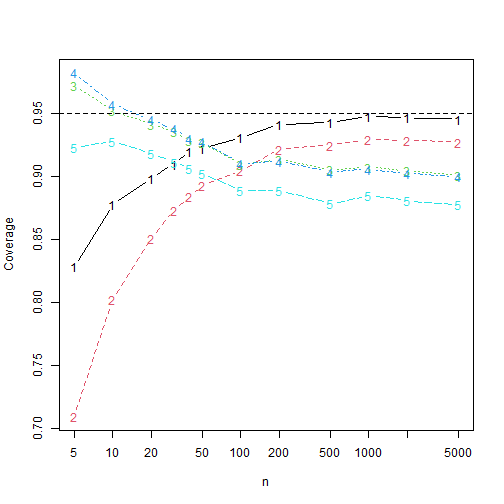# Simulation of confidence intervals.

## Setting up the simulations

We use the dataset bfi from the package psych together with lavaan to estimate some realistic factor loadings $$\lambda$$ and standard deviations $$\sigma$$.

model <- c("y =~ A1 + A2 + A3 + A4 + A5")
fit <- lavaan::cfa(model, data = psych::bfi)
coefs <- lavaan::lavInspect(fit, what = "x")
lambda <- abs(c(coefs$lambda * sqrt(as.numeric(coefs$psi))))
sigma <- sqrt(diag(lavaan::lavInspect(fit, what = "x")\$theta))

We take the absolute value of the lambda vector as the agreement data contains reverse-coded items.

## Comparing confidence intervals coverages and lengths

We compare five confidence intervals, all without transformations. The adf interval is the asymptotic distribution-free interval, the ell interval is the interval based on elliptical distributions and a kurtosis correction, the ell_par is the elliptical interval assuming a parallel model. The same comments hold for norm (assuming normal data) and norm_par (assuming parallel normal data).

library("alphaci")
library("future.apply")
plan(multisession, workers = availableCores() - 2)
set.seed(313)

n_reps <- 10000
k <- 5
latent <- $$n) extraDistr::rlaplace(n) / sqrt(2) true <- alphaci:::alpha(sigma, lambda) In this simulation we normal error terms and a Laplace-distributed latent variable. This one has excess kurtosis \(3$$, which caries over in large part to the data. k is the number of questions ands n_reps is the number of simulations.

success <- $$ci) true <= ci & true >= ci len <- \(ci) ci - ci simulations <- \(n) { results <- future.apply::future_replicate(n_reps, { x <- alphaci:::simulate_congeneric(n, k, sigma, lambda, latent = latent) cis <- rbind(adf = alphaci(x, type = "adf"), adf_par = alphaci(x, type = "adf", parallel = TRUE), ell = alphaci(x, type = "elliptical"), ell_par = alphaci(x, type = "elliptical", parallel = TRUE), norm = alphaci(x, type = "normal"), norm_par = alphaci(x, type = "normal", parallel = TRUE) ) c(cov = apply(cis, 1, success), len = apply(cis, 1, len)) }, future.seed = TRUE) rowMeans(results) } Let’s check out the results when \(n= 10$$.

simulations(10)
#>    0.8745000    0.7942000    0.9513000    0.9566000    0.9223000    0.9297000    0.7680810   55.3239940
#>      len.ell  len.ell_par     len.norm len.norm_par
#>    1.0238478    1.0499270    0.9113473    0.9342908

It appears that the kurtosis corrections work well, at least for small sample size. Let’s see how they perform when $$n$$ increases.

nn <- c(5, 10, 20, 30, 40, 50, 100, 200, 500, 1000, 2000, 5000)
results <- sapply(nn, simulations)

Plotting the coverages, we find, where 1 is asymptotically distribution-free, 2 is elliptical, 3 is paralell and elliptical, 4 is normal and 5 is parallel and normal.

matplot(nn, t(results[1:5, ]), xlab = "n", ylab = "Coverage", type = "b",
log = "x")
abline(h = 0.95, lty = 2)Hence the kurtosis correction intervals have better coverage than the adf interval when $$n\leq 50$$ and outperforms the normal theory intervals for all $$n$$. If this observation is general remains to be seen.